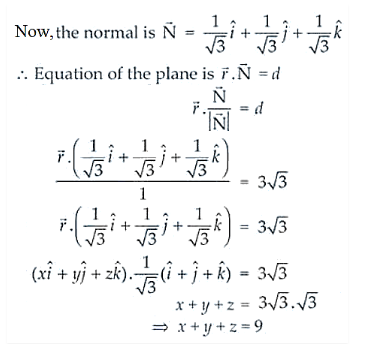# Find the equation of a plane which is at

Question:

Find the equation of a plane which is at a distance 3√3 units from origin and the normal to which is equally inclined to coordinate axis.

Solution:

As the normal to the plane is equally inclined to the axes we have,

cos α = cos β = cos γ

So, cos2 α + cos2 α + cos2 α = 1

3 cos2 α = 1 ⇒ cos α = ± 1/√3

And, cos α = cos β = cos γ = ± 1/√3Thus, the equation of the plane is x + y + z = 9